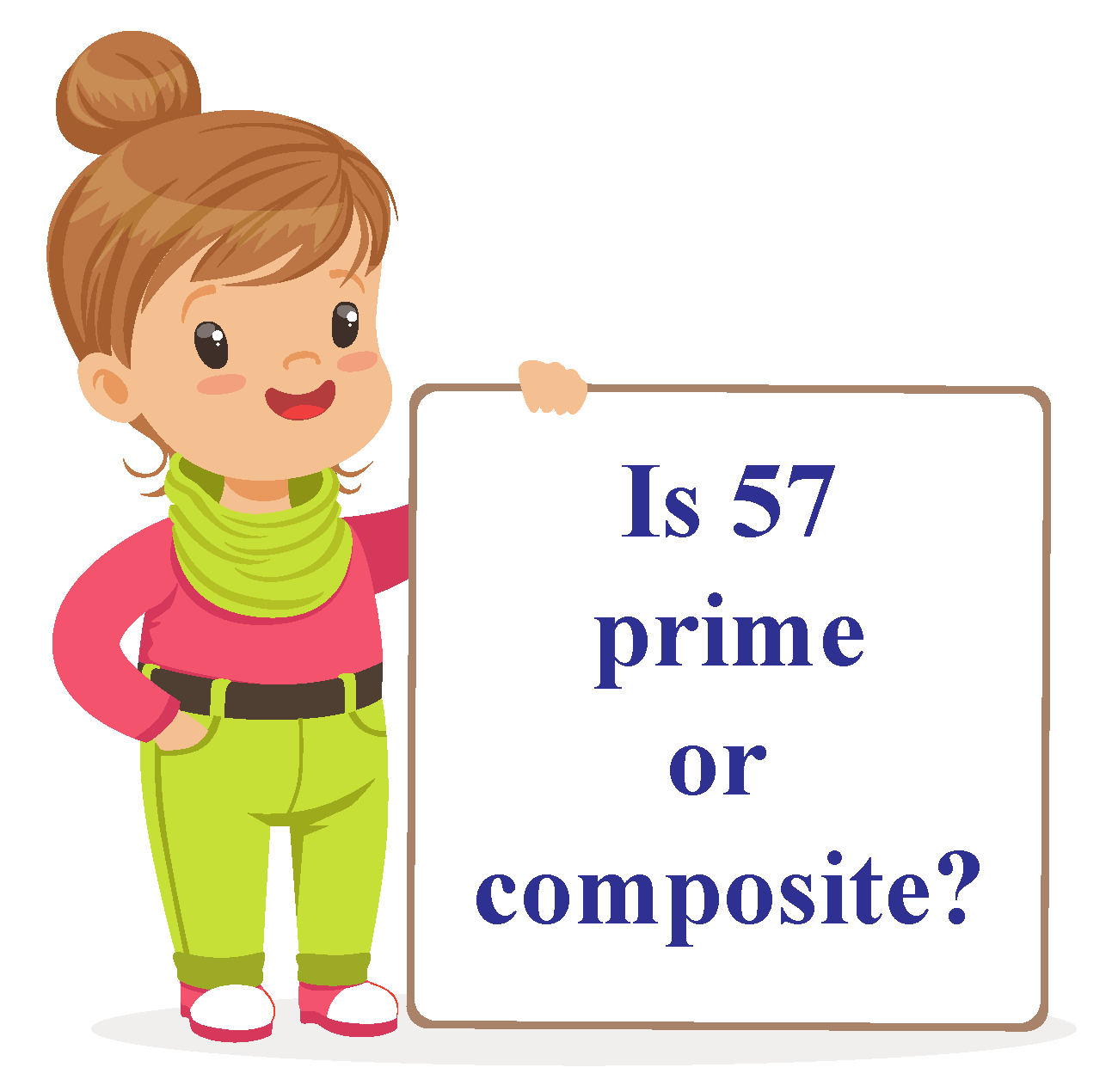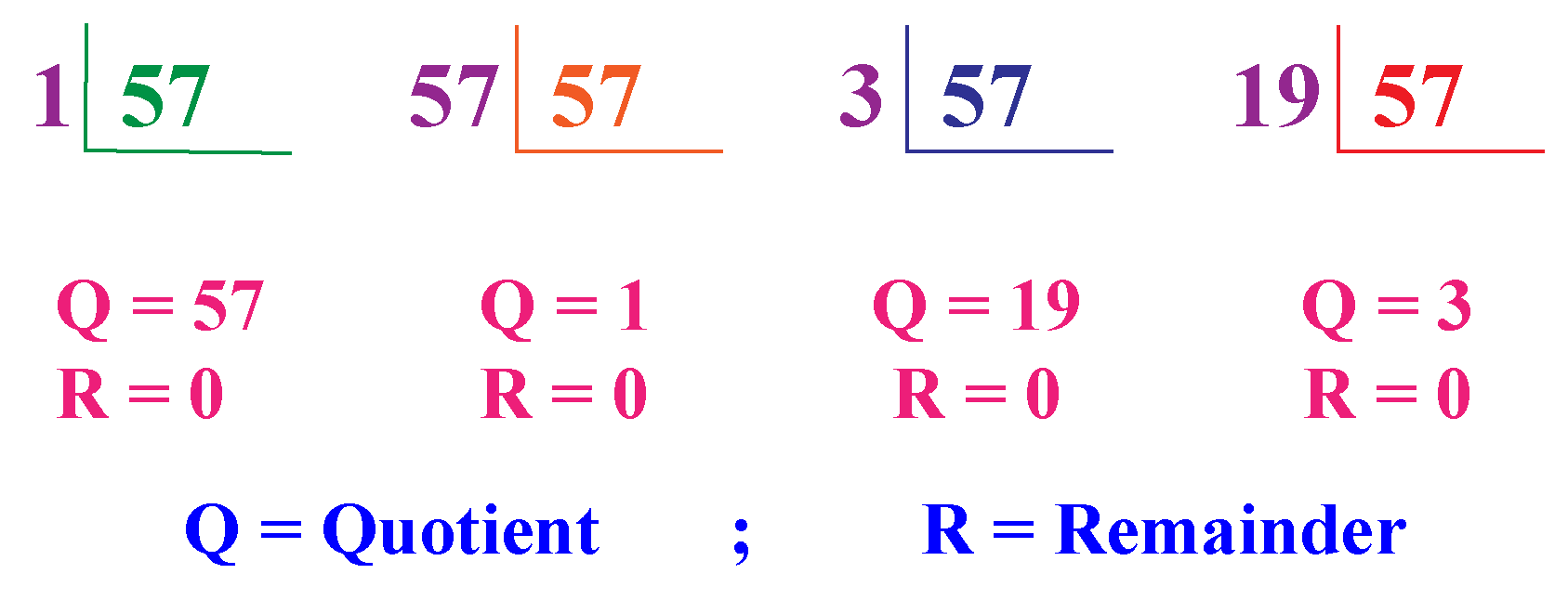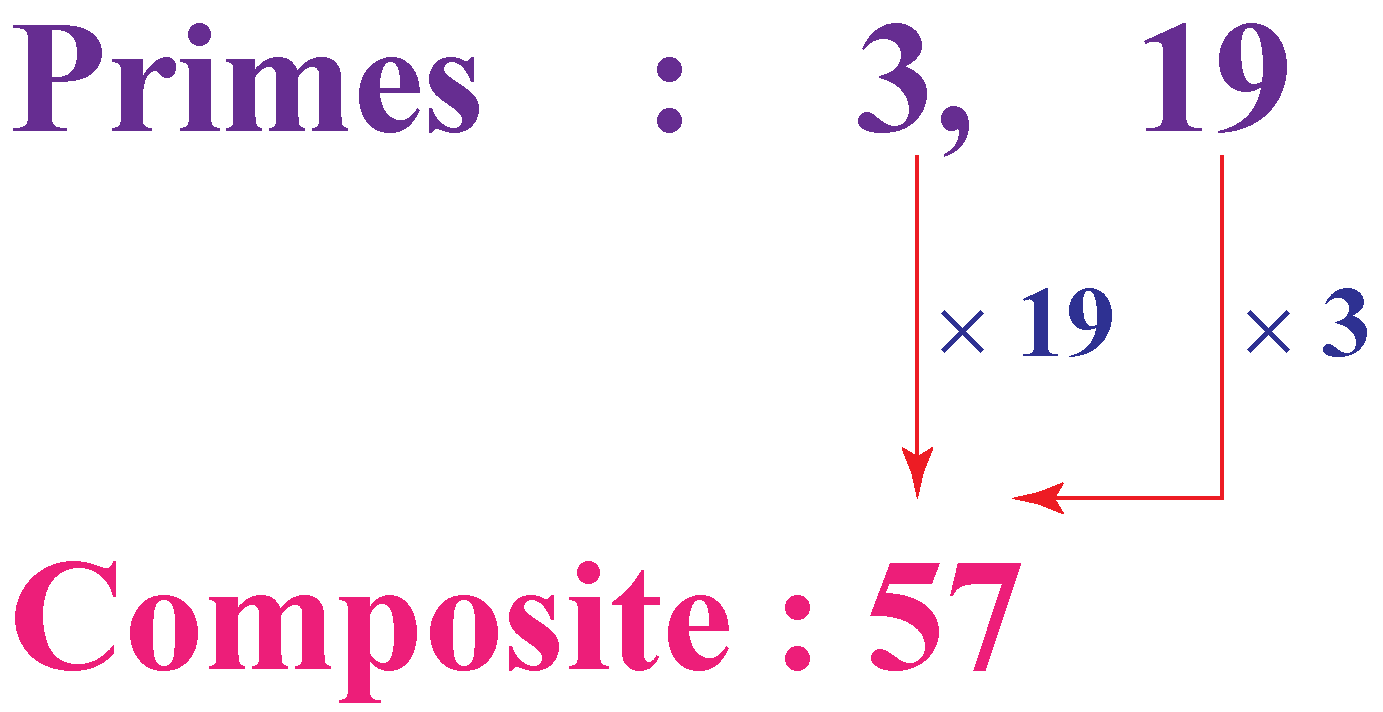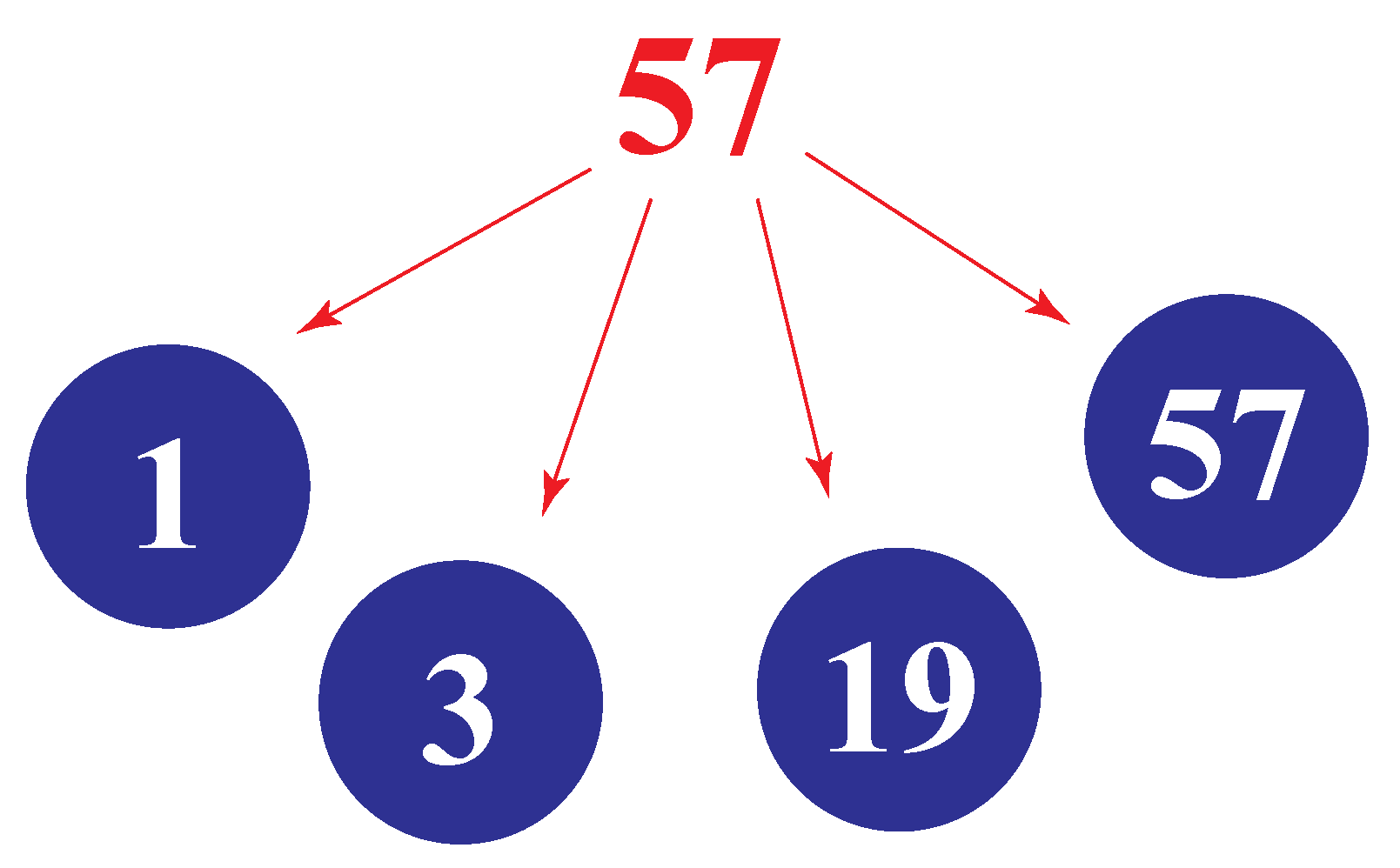# Is 57 a prime number

Is 57 a prime number
Go back to  'Prime Numbers'

Amy's teacher asked her to find the number of ways in which 57 books could be stacked, such that each stack would have equal number of books. She gave the correct answer to her teacher because she knew that 57 has more than two factors, and thus, the books could be arranged in more than two ways!Read on to find how Amy was able to find the answer.

In this mini-lesson, we will learn about prime and composite numbers and find out if 57 is a prime number or a composite number.

## Lesson Plan

 1 Is 57 a Prime Number? 2 Thinking Out of the Box! 3 Important Notes on Is 57 a Prime Number 4 Solved Examples on Is 57 a Prime Number 5 Interactive Questions on Is 57 a Prime Number

## What is a Prime Number?

A whole number that cannot be written as the product of two smaller numbers is called the prime number.

Prime numbers are non-composite numbers.

Examples

Numbers Factors
3 1,3
17 1,17
29 1,29
31 1,31

A prime number is a number that has only 2 factors, 1 and itself.

## Is 57 a Prime Number?

57 is not a prime number because there are four factors of 57

By division method, we find that 1, 3,19, and 57, divide 57 completely without any remainder.

Observe the figure to see that 57 is not a prime number.57 can be expressed as a product of the prime numbers 3 and 19, as shown in the figure.

$$57 = 3\times 19$$Think Tank
• 57 is also called called a semi prime number because it is the product of two prime numbers, 3 and 19

## Is 57 a Composite Number?

57 is a composite number since it has more than two factors.Thus, 57 is composite number, made up of 4 factors 1, 3, 19, 57

## Solved Examples

 Example 1

Mary has three number cards with 3, 19 and 57 printed on them respectively. She needs to pick the card which has a prime number. Can u help her?

Solution

Mary knows that 3 has only two factors, 1 and 3

Similarly, 19 has only two factors, 1 and 19

However, 57 can be factored as $$57=1\times 57$$ and $$57=3\times 19$$

Thus, she found that 57 is not a prime number, while 3 and 19 are prime numbers.

 $$\therefore$$ 3 and 19 are the prime numbers.
 Example 2

Three friends collected 57 sea shells on the seashore. How will they distribute these shells among themselves equally?

Solution

$$3 \times \text{____}= 57$$

$$57\div 3 = 19$$

The missing factor is 19

 $$\therefore$$ Each friend will get 19 shells.

## Interactive Questions

Here are a few activities for you to practice. Select/Type your answer and click the "Check Answer" button to see the result.

## Let's Summarize

The mini-lesson targeted on the concept to check if 57 is a prime number. The proof that 57 is not a prime number is verified by many examples and illustrations. Now, you will be able to easily solve problems on prime numbers and composite numbers.Done in a way that not only it is relatable and easy to grasp, but also will stay with them forever. Here lies the magic with Cuemath.

At Cuemath, our team of math experts is dedicated to making learning fun for our favorite readers, the students!

Through an interactive and engaging learning-teaching-learning approach, the teachers explore all angles of a topic.

Be it worksheets, online classes, doubt sessions, or any other form of relation, it’s the logical thinking and smart learning approach that we, at Cuemath, believe in.

## 1. What is a coprime number?

Two numbers are co-prime if they have no common factors other than 1

5 and 9 are the numbers which have only 1 as their common factor. Therefore, 5 and 9 are co-prime numbers.

## 2. What are the factors of 57?

The factors of 57 are 1, 3, 19 and 57

## 3. What is the prime factorization of 57?

The prime factorization of 57 is $$3\times 19$$

## 4. Are 52 and 57 prime numbers?

No, 52 and 57 are not prime numbers since they have more than 2 factors. They are composite numbers.

More Important Topics
Numbers
Algebra
Geometry
Measurement
Money
Data
Trigonometry
Calculus